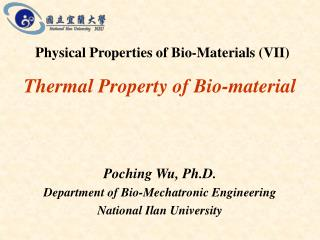DownloadDownload PresentationThermal Property of Bio-material

# Thermal Property of Bio-material

Download Presentation## Thermal Property of Bio-material

- - - - - - - - - - - - - - - - - - - - - - - - - - - E N D - - - - - - - - - - - - - - - - - - - - - - - - - - -
##### Presentation Transcript

1. Thermal Property of Bio-material Physical Properties of Bio-Materials (VII) Poching Wu, Ph.D. Department of Bio-Mechatronic Engineering National Ilan University

2. Thermal Properties of Bio-material • Dimensional Characteristics: Shape, Size, Volume, Roundness, Sphericity, Unit Surface Area, Average Projected area • Density • Fluid Viscosity • Unit Surface Conductance • Latent Heat

3. Thermal Properties of Bio-material • Specific Heat • Thermal Conductivity • Mass Diffusivity or Diffusion Coefficient • Mass Transfer Coefficient • Coefficient of Thermal Expansion • Dimensionless Parameters

4. Specific Heat where • C = specific heat, kJ/kg·℃ • Q = the heat supplied, kJ • w = specific weight, kg/m3 • V = volume, m3 • m = mass, kg • Dt = Temperature Difference, ℃

5. The specific heat of a substance denotes the variation of the temperature with the amount of heat stored within the substance. • This equation indicates that C is also a function of temperature.

6. Measurement of Specific Heat • Siebel’s Equations • Method of Mixture • Method of Guarded-Plate • Method of Comparison Calorimeter • Method of Calculated Specific Heat • Method of Differential Scanning Calorimetry (DSC)

7. Siebel’s Equations (1892) For values above freezing, For values below freezing, where C = specific heat, BTU/lb·ºF M = moisture content of the material in percent wet basis, %. 0.2 is a constant assumed to be the specific heat of the solid. ※ 1 BTU/lb·ºF = 4.187 kJ/kg·℃

8. Haswell (1954) Rough rice Finished rice Oats

9. Method of Mixture

10. Method of Mixture where Cs = specific heat of sample, kJ/kg·℃ Cw = specific heat of water, 4.2 kJ/kg·℃ Cc = specific heat of calorimeter cup or bucket, kJ/kg·℃ Ww = weight of added water, kg Wc = weight of calorimeter cup or bucket, kg Ws = weight of sample, kg te = equilibrium temperature,℃ tw = initial water temperature,℃ ti = initial temperature of sample and calorimeter cup or bucket,℃

11. The accuracy of this method is based on the assumption that the unaccounted-for heat losses are negligible. Example: Cw = specific heat of water, 4.187 kJ/kg·℃ Cc = specific heat of calorimeter cup or bucket, 0.946 kJ/kg·℃ Ww = weight of added water, 0.254 kg Wc = weight of calorimeter cup or bucket, 0.054 kg Ws = weight of sample, 0.090 kg te = equilibrium temperature, 30℃ tw = initial water temperature, 21℃ ti = initial temperature of sample and calorimeter cup or bucket, 73℃ substituting the given information in the equation listed above yields Cs = 1.842 kJ/kg·℃

12. Correction for Thermal Leakage

13. Method of Guarded-Plate

14. Method of Guarded-Plate where V = average voltage I = average current Θ = time, sec 3.41 = the conversion factor from watts to BTU/hr

15. Method of Comparison Calorimeter

17. Method of Differential Scanning Calorimetry

18. Thermal ConductionFourier’s Law of Heat Conduction • k = thermal conductivity, W/m℃ • A = cross section area, m2 • dT/dx = temperature gradient, ℃/m

19. Factors affect the thermal conductivity • Temperature • the state of the substance • chemical composition • Physical (Cellular) structure • Density • Moisture Content • Moisture migration Heat conduction is usually interpreted either as molecular interchange of kinetic energy or electron drift (the mobility of free electrons)

20. Measurement of Thermal Conductivity Steady-State • Longitudinal Heat Flow Methods • Radial Heat Flow Methods Cylinder Without End Guards Cylinder With End Guards Sphere With Central Heating Source Concentric Cylinder Comparative Method • Heat of Vaporization Methods

21. Measurement of Thermal Conductivity Unsteady-State • Fitch Method • Line Heat Source Method • Plane Heat Source Method • Statistical Modeling Method • Frequency Response Method • Packed Bed Analysis Method

22. Heat Conduction EquationIn a Large Plane Wall Steady State: Transient:

23. Longitudinal Heat Flow Methods • k = thermal conductivity, W/m℃ • d = specimen thickness, m • q = measured rate of heat input, W • A = area of specimen, m2 • Dt = temperature difference between specimen surfaces normal to heat flow, ℃